Use All 4 Quadrants of the Coordinate Plane Math Video - Grades 6-8
1%
It was processed successfully!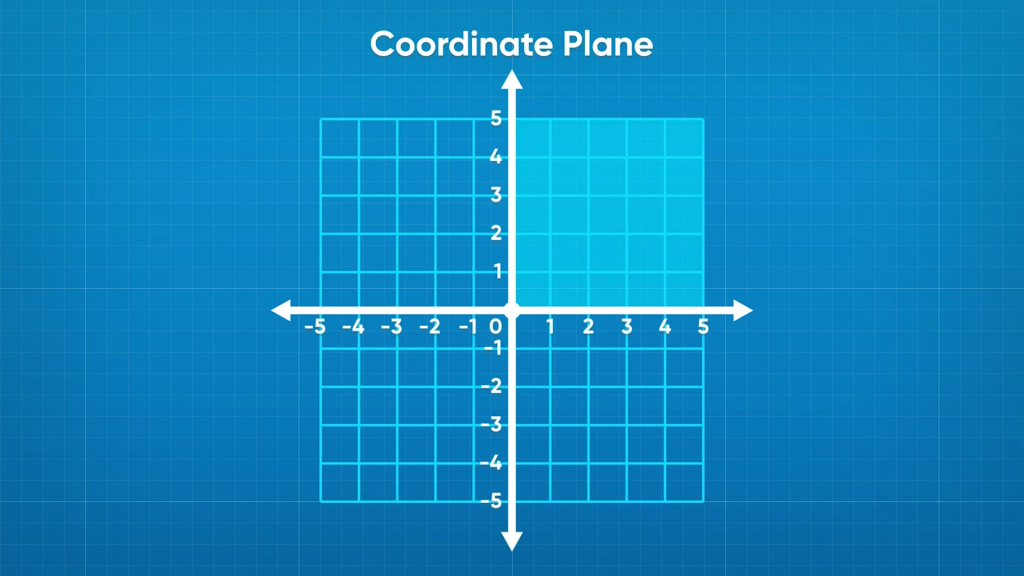THE COORDINATE PLANE HAS 4 QUADRANTS.

A number line goes on infinitely in both directions; so do the axes on a coordinate grid. Up until now, you only plotted points in the section of the coordinate plane where both x– and y-values were positive. You can also plot points where either value (or both!) is negative.

To better understand using all 4 quadrants of the coordinate plane…

THE COORDINATE PLANE HAS 4 QUADRANTS. A number line goes on infinitely in both directions; so do the axes on a coordinate grid. Up until now, you only plotted points in the section of the coordinate plane where both x– and y-values were positive. You can also plot points where either value (or both!) is negative. To better understand using all 4 quadrants of the coordinate plane…

## LET’S BREAK IT DOWN!

### Expanding the coordinate grid.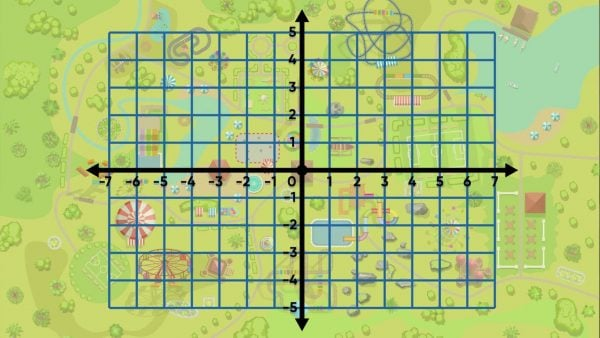An amusement park uses a coordinate grid to map out locations for rides. The coordinate grid shows new numbers now: the y-axis extends past 0 to the negative numbers, and so does the x-axis. There are now four sections, or quadrants, on the grid. Try this yourself: Identify the quadrant on the coordinate grid where you have plotted points before.

Expanding the coordinate grid. An amusement park uses a coordinate grid to map out locations for rides. The coordinate grid shows new numbers now: the y-axis extends past 0 to the negative numbers, and so does the x-axis. There are now four sections, or quadrants, on the grid. Try this yourself: Identify the quadrant on the coordinate grid where you have plotted points before.

### Plot points in all four quadrants in the coordinate grid.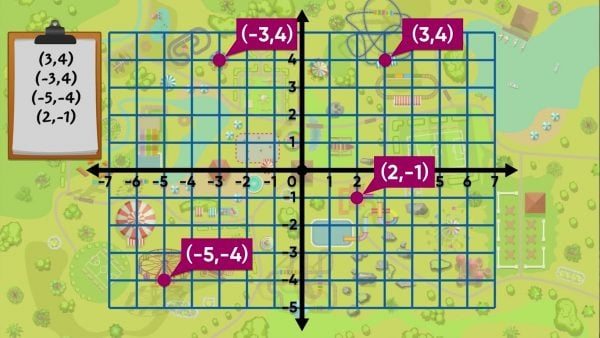The locations of four rides at the park are: Radical Rollercoaster (3, 4), Elevator Doom Drop (−3, 4), Tilt-A-Whirl (−5, −4), and Raging Rapids Raft Adventure (2, −1). To locate the Radical Rollercoaster, start at the origin and go right 3 units on the x-axis to 3. Then move up 4 to the point (3, 4). To locate the Doom Drop Tower, move 3 units to the left of 0 on the x-axis to −3. Next, move up 4 units from 0 on the y-axis to 4. To locate the Tilt-A-Whirl, move left from 0 on the x-axis 5 units and down 4 units below 0 on the y-axis to the point (−5, −4). To locate Raging Rapids, at point (2, −1), move 2 units to the right of 0 on the x-axis and 1 unit below 0 on the y-axis. Try this yourself: Describe how you would plot the Gift Shop at (−2, −2).

Plot points in all four quadrants in the coordinate grid. The locations of four rides at the park are: Radical Rollercoaster (3, 4), Elevator Doom Drop (−3, 4), Tilt-A-Whirl (−5, −4), and Raging Rapids Raft Adventure (2, −1). To locate the Radical Rollercoaster, start at the origin and go right 3 units on the x-axis to 3. Then move up 4 to the point (3, 4). To locate the Doom Drop Tower, move 3 units to the left of 0 on the x-axis to −3. Next, move up 4 units from 0 on the y-axis to 4. To locate the Tilt-A-Whirl, move left from 0 on the x-axis 5 units and down 4 units below 0 on the y-axis to the point (−5, −4). To locate Raging Rapids, at point (2, −1), move 2 units to the right of 0 on the x-axis and 1 unit below 0 on the y-axis. Try this yourself: Describe how you would plot the Gift Shop at (−2, −2).

### Draw and measure lines on the coordinate grid.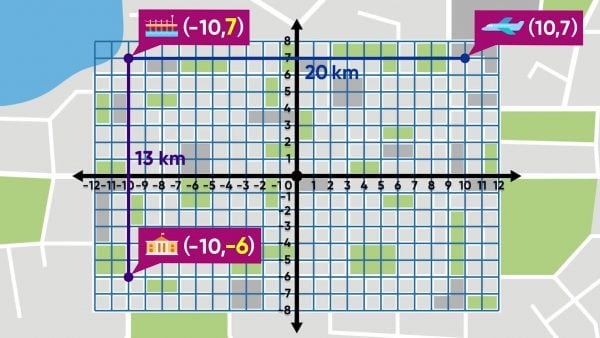You need to find the distance between two locations on a city map. The pier is at (−10, 7) and the airport is at (10, 7). Both landmarks have the same y-coordinate, so they lie on a horizontal line. The distance is the number of units from one x-coordinate to the other. From −10 to 10 is 20 units. If each unit is 1 kilometer, the distance between these locations is 20 kilometers. You can also subtract the x-coordinates to find the distance: 10--10=20. To find the distance from the pier at point (−10, 7) and City Hall at point (−10, −6), subtract the y-coordinates: 7--6 = 13. The Pier and City Hall are 13 kilometers apart. Try this yourself: What is the distance between the Art Museum at (4, −6) and the Hotel at (4, −2)?

Draw and measure lines on the coordinate grid. You need to find the distance between two locations on a city map. The pier is at (−10, 7) and the airport is at (10, 7). Both landmarks have the same y-coordinate, so they lie on a horizontal line. The distance is the number of units from one x-coordinate to the other. From −10 to 10 is 20 units. If each unit is 1 kilometer, the distance between these locations is 20 kilometers. You can also subtract the x-coordinates to find the distance: 10--10=20. To find the distance from the pier at point (−10, 7) and City Hall at point (−10, −6), subtract the y-coordinates: 7--6 = 13. The Pier and City Hall are 13 kilometers apart. Try this yourself: What is the distance between the Art Museum at (4, −6) and the Hotel at (4, −2)?

### Find the perimeter of a shape using coordinates.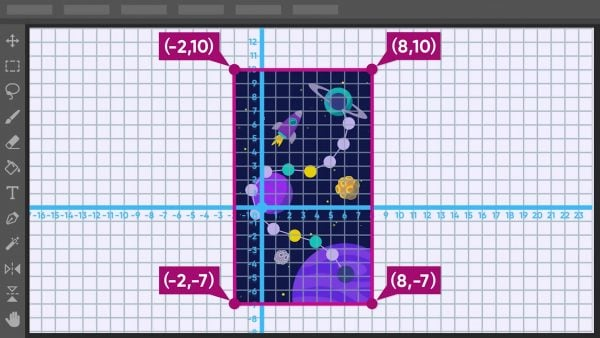You’ve designed a board game on a coordinate grid on the computer. The gameboard is a rectangle with vertices at (8, 10), (8, −7), (−2, −7), and (−2, 10). You need to calculate the area and perimeter of the gameboard. First, find the side lengths of the rectangle. Find the vertical distance between (8, 10) and (8, −7): 10-(-7) = 17. One side length is 17 units. Next, find the horizontal distance between the points (8, –7) and (–2, –7): 8-(-2) = 10. The other side length is 10 units. On a rectangle, opposite sides have equal length. The perimeter is the sum 17+10+17+10=54 units. If 1 unit is equal to 1 centimeter, the board game has perimeter 54 centimeters. The area of the rectangle is length times width, or 17 × 10=170 square centimeters. Try this yourself: What is the perimeter of a rectangle with vertices at (2, 2), (−2, 2), (−2, −2) and (2, −2)?

Find the perimeter of a shape using coordinates. You’ve designed a board game on a coordinate grid on the computer. The gameboard is a rectangle with vertices at (8, 10), (8, −7), (−2, −7), and (−2, 10). You need to calculate the area and perimeter of the gameboard. First, find the side lengths of the rectangle. Find the vertical distance between (8, 10) and (8, −7): 10-(-7) = 17. One side length is 17 units. Next, find the horizontal distance between the points (8, –7) and (–2, –7): 8-(-2) = 10. The other side length is 10 units. On a rectangle, opposite sides have equal length. The perimeter is the sum 17+10+17+10=54 units. If 1 unit is equal to 1 centimeter, the board game has perimeter 54 centimeters. The area of the rectangle is length times width, or 17 × 10=170 square centimeters. Try this yourself: What is the perimeter of a rectangle with vertices at (2, 2), (−2, 2), (−2, −2) and (2, −2)?

## USE ALL 4 QUADRANTS OF THE COORDINATE PLANE VOCABULARY

Coordinate plane
A grid that contains two number lines that intersect at a right angle at 0 and divide the plane into four quadrants.
Origin
The point where the two axes of a coordinate grid intersect; the point is represented by the ordered pair (0, 0).
Ordered pair
The location of a point on a coordinate plane written as (x, y).
x-axis
The horizontal number line on a coordinate grid.
y-axis
The vertical number line on a coordinate grid.
x-coordinate
The first number in an ordered pair, which names the distance to the right or left from the origin along the x-axis.
The second number in an ordered pair, which names the distance up or down from the origin along the y-axis.
The distance a number is from 0 on the number line.

## USE ALL 4 QUADRANTS OF THE COORDINATE PLANE DISCUSSION QUESTIONS

### Describe how to locate the point (–9, 7) on the coordinate grid.

Begin at the origin, move left 9 units on the x-axis, and then move up 7 units on the y-axis.

### What are two different ways to find the length of a line segment between points (–3, 5) and (6, 5)?

Graph the two points on a coordinate plane and count the number of units between the x-coordinates (add the absolute values), or subtract the smaller x-coordinate from the larger x-coordinate (6 – (–3) = 9).

(–1, 1)

A vertical line

28 square units
X

## Success

We’ve sent you an email with instructions how to reset your password.
Ok